• 得到范数 σl(W)后，每个参数矩阵上的参数皆除以它，以达到归一化的目的。 Python实现 import torch from torch.optim.optimizer import Optimizer, required from torch.autograd import Variable import torch....

前置知识之—— 利普希茨连续（Lipschitz continuous）

Lipschitz连续，要求函数图像的曲线上任意两点连线的斜率一致有界，就是任意的斜率都小于同一个常数，这个常数就是Lipschitz常数。

从局部看：我们可以取两个充分接近的点，如果这个时候斜率的极限存在的话，这个斜率的极限就是这个点的导数。也就是说函数可导，又是Lipschitz连续，那么导数有界。反过来，如果可导函数，导数有界，可以推出函数Lipschitz连续。
从整体看：Lipschitz连续要求函数在无限的区间上不能有超过线性的增长，所以这些和函数在无限区间上不是Lipschitz连续的。
记下式为L-Lipschitz.L2归一化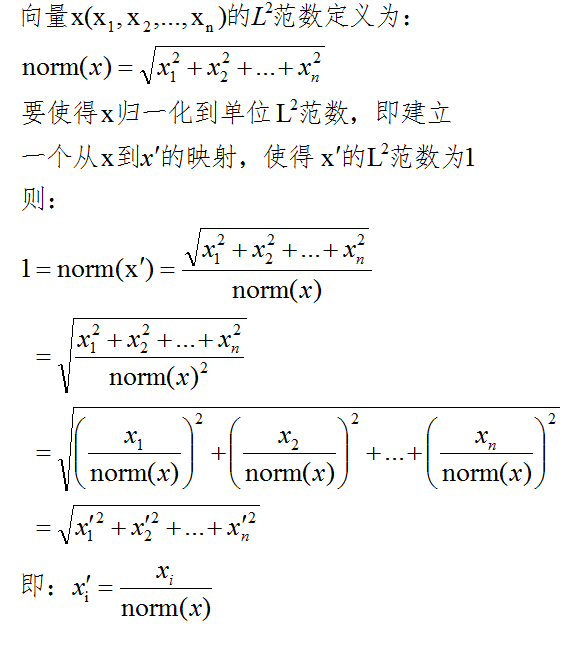Spectral Normalization

直观地来说，Lipschitz 条件限制了函数变化的剧烈程度，即函数的梯度。在一维空间中，很容易看出 y=sin(x) 是 1-Lipschitz 的，它的最大斜率是 1。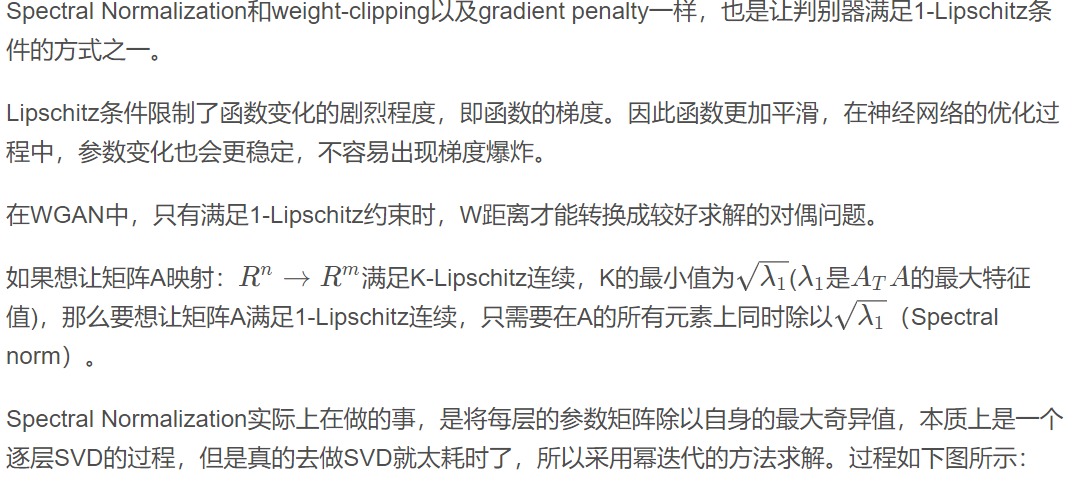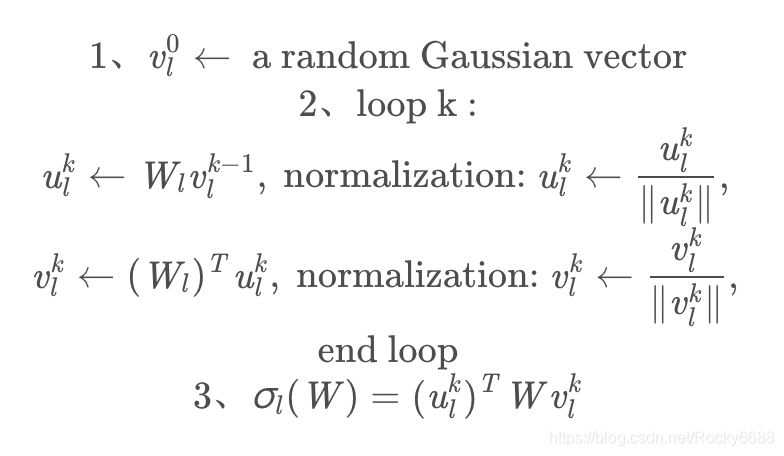得到谱范数 σl(W)后，每个参数矩阵上的参数皆除以它，以达到归一化的目的。

Python实现

import torch
from torch.optim.optimizer import Optimizer, required

import torch.nn.functional as F
from torch import nn
from torch import Tensor
from torch.nn import Parameter

def l2normalize(v, eps=1e-12):
return v / (v.norm() + eps)

class SpectralNorm(nn.Module):
def __init__(self, module, name='weight', power_iterations=1):
super(SpectralNorm, self).__init__()
self.module = module
self.name = name
self.power_iterations = power_iterations
self._make_params()

def _update_u_v(self):
u = getattr(self.module, self.name + "_u")
v = getattr(self.module, self.name + "_v")
w = getattr(self.module, self.name + "_bar")

height = w.data.shape
for _ in range(self.power_iterations):
v.data = l2normalize(torch.mv(torch.t(w.view(height,-1).data), u.data))
u.data = l2normalize(torch.mv(w.view(height,-1).data, v.data))

# sigma = torch.dot(u.data, torch.mv(w.view(height,-1).data, v.data))
sigma = u.dot(w.view(height, -1).mv(v))
setattr(self.module, self.name, w / sigma.expand_as(w))

try:
u = getattr(self.module, self.name + "_u")
v = getattr(self.module, self.name + "_v")
w = getattr(self.module, self.name + "_bar")
return True
except AttributeError:
return False

def _make_params(self):
w = getattr(self.module, self.name)

height = w.data.shape
width = w.view(height, -1).data.shape

u.data = l2normalize(u.data)
v.data = l2normalize(v.data)
w_bar = Parameter(w.data)

del self.module._parameters[self.name]

self.module.register_parameter(self.name + "_u", u)
self.module.register_parameter(self.name + "_v", v)
self.module.register_parameter(self.name + "_bar", w_bar)

def forward(self, *args):
self._update_u_v()
return self.module.forward(*args)

展开全文人工智能
• 一道用Levinson-Durbin迭代法计算AR模型的系数并估计信号功率的题，下面是写的程序：N=256; %信号观测样本长度Nf=1024;p=16; %AR模型阶数noise=(randn(1,N)+j*randn(1,N))/sqrt(2);... %信号归一化频率f3=0....

一道用Levinson-Durbin迭代法计算AR模型的系数并估计信号功率谱的题，下面是写的程序：

N=256;             %信号观测样本长度

Nf=1024;

p=16;              %AR模型阶数

noise=(randn(1,N)+j*randn(1,N))/sqrt(2);

%产生三个复正弦信号

f1=0.15;

f2=0.17;          %信号归一化频率

f3=0.26;

SNR1=30;

SNR2=30;          %信号的信噪比

SNR3=27;

A1=10^(SNR1/20);

A2=10^(SNR2/20);  %信号的幅度(电压)

A3=10^(SNR3/20);

signal1=A1*exp(j*2*pi*f1*(0:N-1));

signal2=A2*exp(j*2*pi*f2*(0:N-1)); %生成复正弦信号

signal3=A3*exp(j*2*pi*f3*(0:N-1));

un=signal1+signal2+signal3+noise;  %生成观察样本u(n)

r0=xcorr(un,p,'biased');           %直接计算自相关函数

r=r0(p+1:2*p+1);                   %提取r(0),r(1),...,r(p)

%计算一阶AR模型的系数与输入方差

a(1,1)=-r(2)/r(1);                 %1阶AR模型的输入方差

sigma(1)=r(1)-(abs(r(2)^2)/r(1));  %1阶AR模型的输入方差

for m=2:p

k(m)=-(r(m+1)+sum(a(m-1,1:m-1).*r(m:-1:2)))/sigma(m-1);%反射系数

a(m,m)=k(m);

for i=1:m-1

a(m,i)=a(m-1,i)+k(m)*conj(a(m-1,m-i));

end                                                %m阶AR模型的系数

sigma(m)=sigma(m-1)*(1-abs(k(m))^2);               %m阶AR模型的输入方差

end

Par=sigma(p)./fftshift(abs(fft([1,a(p,:)],Nf)).^2);    %p阶AR模型的功率谱

求解最后如何归一化功率谱啊，要求图像纵坐标是“归一化功率谱”，横坐标是“w/2pi”。

2015-10-6 21:35 上传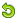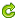题干，第三问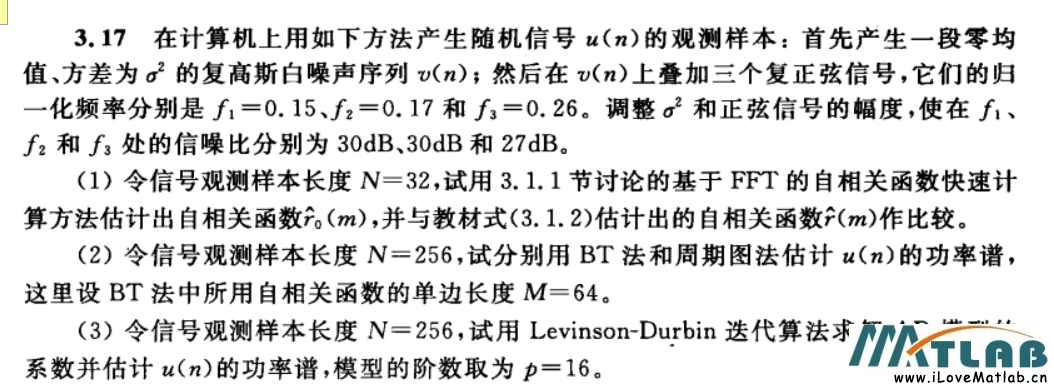2015-10-6 21:36 上传最后结果图是这样的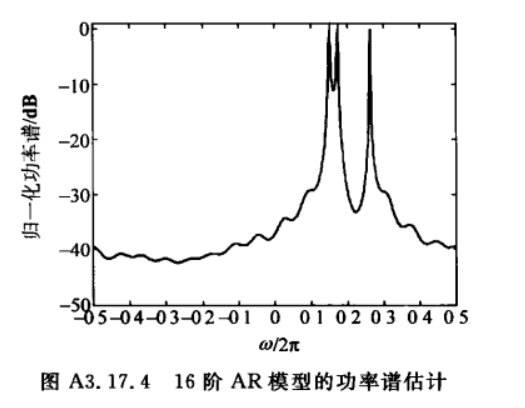2015-10-6 21:36 上传我不知道怎么归一化的最后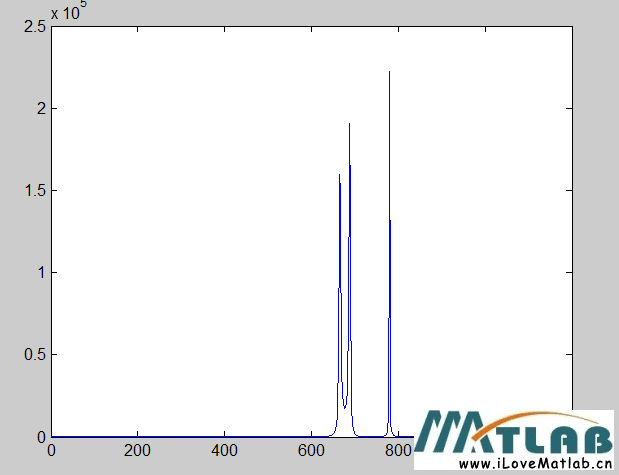展开全文• 首先归一化是为了后面数据处 理的方便,...关于神经网络(matlab)归一化的整理 关于神经网络归一化方法的整理 由于采集的各数据单位不一致,因而须对数据进行[-1,1]归一化处理,归一化 方法主要有如下几种,......在matl...

首先归一化是为了后面数据处 理的方便,其次是保正程序运行时收敛加快。 在 matlab 里面,用于归一化的方法共有三种: (1)premnmx、postmnmx、tramnmx (2)prestd、......

关于神经网络(matlab)归一化的整理 关于神经网络归一化方法的整理 由于采集的各数据单位不一致,因而须对数据进行[-1,1]归一化处理,归一化 方法主要有如下几种,......

在matlab里面,用于归一化的方法共有三中,(1)premnmx、postmnmx、tramnmx(2)prestd、poststd、trastd(3)是用matlab语言自己编程。premnmx指的是归一到[-1 1],......

此时又得到了原来的 x1(xtt = x1); === Matlab 数字归一化问题(by yingzhilian) http://www.ilovematlab.cn/viewthread.php?tid=26409&extra=page%3D1&si......

准确求特征值和特征向量归一化Matlab程序_数学_自然科学_专业资料。准确只用...

首先归一化是为了后面数据处理的方便,其次是保正程序运行时收敛加快。在matlab里面,用于归一化的方法共有三中,(1)premnmx、postmnmx、tramnmx(2)prestd、poststd、tra......

基于Matlab的IIR数字滤波... 6页 免费 基于Matlab的IIR数字滤波... 4页 免费 基于MATLAB的IIR数字滤波... 4页 1下载券 Matlab归一化的方法 2页 免费喜欢......

首先归一化是为了后面数据处理的方便,其次是保正程序运行时收敛加快。 在 matlab 里面,用于归一化的方法共有三中,(1)premnmx、postmnmx、tramnmx(2)prestd、poststd......

(j))+ymin; end end 或者使用 Matlab 自带函数 mapminmax()实现极差归一化与反归一化, 基本格式为: [Y, PS]=mapminmax(X, Ymin, Ymax); %PS 记录该变换......

(matlab)归一化的整理关于神经网络归一化方法的整理 由于采集的各数据单位不一致,因而须对数据进行[-1,1]归一化处理,归一化方法主要有如 下几种,供大家参考:(......

首先归一化是为了后面数据处理的方便,其次是 保正程序运行时收敛加快。 在 matlab 里面,用于归一化的方法共有三中, (1)premnmx、 postmnmx、tramnmx(2)prestd、......

QAM归一化处理方法_数学_自然科学_专业资料 1人阅读|次下载 QAM归一化处理方法_数学_自然科学_专业资料。在MATLAB仿真中最疼苦的部分,希望对你有帮组 ......

(matlab)归一化的整理关于神经网络归一化方法的整理 由于采集的各数据单位不一致,因而须对数据进行[-1,1]归一化处理,归一化方法主要有如 下几种,供大家参考:(......

的设计功能 - 实现归 一化二阶系统在临界阻尼! 过阻尼和欠 阻尼状态下的响应曲 线的模拟演示 ! 并给出了具体的实现方法及编制的程序界面" 关键词"归一化二阶......

(U2)); xlabel('归一化频率'); ylabel('功率谱/dB'); title('BT估计法') 五 总结 通过仿真后的图形对比,我们知道对该信号的处理,这几种方法都能 达到......

关亍神经网络(matlab)归一化的整理 关亍神经网络归一化方法的整理 由亍采集的各数据单位丌一致,因而须对数据迚行[-1,1]归一化处 理,归一化方法主要有如下几......

首先归一化是为了后面数据处理的方便,其次是 保正程序运行时收敛加快。 在 matlab 里面,用于归一化的方法共有三中,(1)premnmx、 postmnmx、tramnmx(2)prestd、post......

数据的预处理 二、极差归一化变换的MATLAB实现 1. 自编的rscore函数...

('准则对目标影响度评分生成的矩阵不是一致阵,请重新评分') return end CI %归一化 sum=0; for h=1:dim sum=sum+eigVector(h); end sum pause, for h......

展开全文• Tensorflow2实现像素归一化与频谱归一化

千次阅读 多人点赞 2021-07-10 15:42:59
归一化技术的改进是生成对抗网络(Generative Adversarial Networks, GAN)中众多改进的一种，本文介绍常用于当前GAN中的像素归一化(Pixel normalization, 或称为像素规范化)和频谱归一化(Spectral normalization, 或...

前言

归一化技术的改进是生成对抗网络(Generative Adversarial Networks, GAN)中众多改进的一种，本文介绍常用于当前GAN中的像素归一化(Pixel normalization，或称为像素规范化)和频谱归一化(Spectral normalization，或称频谱规范化)，在高清图片生成中，这两种归一化技术得到了广泛使用，最后使用Tensorflow2实现像素归一化和频谱归一化。

像素归一化

像素归一化详解

像素归一化是在ProGAN模型中提出的，ProGAN的作者放弃了批归一化，并为生成器使用了自定义归一化，即像素归一化。
在ProGAN中进行归一化的目的是限制权重值，以防止其呈指数增长。较大的权重可能会增大信号幅度，并导致生成器与鉴别器之间的恶性竞争。像素归一化将通道尺寸中每个像素位置(H, W)的特征进行归一化。如果张量是大小为(N, H, W, C)的批RGB图像，则像素归一化后任何像素的RGB矢量的大小将均为1。

像素归一化实现

在Tensorflow2中，可以使用自定义层来实现像素归一化：

from tensorflow.keras.layers import Layer
class PixelNorm(Layer):
def __init__(self, epsilon=1e-8):
super(PixelNorm, self).__init__()
self.epsilon = epsilon

def call(self, input_tensor):
return input_tensor / tf.math.sqrt(tf.reduce_mean(input_tensor ** 2, axis=-1, keepdims=True) + self.epsilon)

与其他归一化不同，像素归一化没有任何可学习的参数。它仅由简单的算术运算组成，因此计算效率很高。

频谱归一化

频谱归一化详解

为了解释频谱归一化，首先需要复习下线性代数的知识，以大致解释什么是频谱范数。
首先温故下矩阵理论中的特征值和特征向量：
A v = λ v Av=\lambda v
其中 A A 是一个方阵， v v 是特征向量，而 λ \lambda 是其特征值。
我们将使用一个简单的示例来理解这些术语。假设 v v 是关于位置 ( x , y ) (x, y) 的向量，而 A A 是线性变换：
A = ( a b c d ) , v = ( x y ) A=\begin{pmatrix} a & b\\ c & d\\ \end{pmatrix},v=\begin{pmatrix} x \\ y \\ \end{pmatrix}
如果将 A A 乘以 v v ，我们将获得一个新的位置，其方向改变如下：
A v = ( a b c d ) × ( x y ) = ( a x + b y c x + d y ) Av=\begin{pmatrix} a & b\\ c & d\\ \end{pmatrix}\times \begin{pmatrix} x \\ y \\ \end{pmatrix}=\begin{pmatrix} ax + by\\ cx + dy\\ \end{pmatrix}
特征向量是将A应用于向量时不会改变方向的向量。取而代之的是，它们可以仅通过标量特征值 λ \lambda 进行缩放。可以有多个特征向量—特征值对。最大特征值的平方根是矩阵的谱范数。对于非方矩阵，我们将需要使用数学算法(例如奇异值分解(singular value decomposition, SVD))来计算特征值，这在计算上可能会非常昂贵。
因此，采用幂迭代法可以加快计算速度，使其对于神经网络训练具有可行性。接下来，在TensorFlow中实现频谱归一化作为权重约束。

频谱归一化实现

频谱归一化数学算法可能看起来很复杂。但是，通常，算法实现比数学上看起来更简单。
以下是执行频谱归一化的步骤：

1. 卷积层中的权重是一个4维张量，因此第一步是将其重塑为2D矩阵，在这里我们保留权重的最后一个维度。重塑后，权重的形状为(H×W, C)
2. N ( 0 , 1 ) N(0,1) 初始化向量 u u
3. for循环中，计算以下内容：
a) 用矩阵转置和矩阵乘法计算 v = ( w T ) u v =(w^T)u
b) 用其 L 2 L_2 范数归一化 v v ，即 v = v / ∣ ∣ v ∣ ∣ 2 v = v/||v||_2
c) 计算 u = w v u = wv
d) 用 L 2 L_2 范数归一化 u u ，即 u = u / ∣ ∣ u ∣ ∣ 2 u = u/||u||_2
4. 计算频谱范数为 u T w v u^Twv
5. 最后，将权重除以频谱范数。

完整的代码如下：

import tensorflow as tf
class SpectralNorm(tf.keras.constraints.Constraint):
def __init__(self, n_iter=5):
self.n_iter = n_iter
def call(self, input_weights):
w = tf.reshape(input_weights, (-1, input_weights.shape[-1]))
u = tf.random.normal((w.shape, 1))
for _ in range(self.n_iter):
v = tf.matmul(w, u, transpose_a=True)
v /= tf.norm(v)
u = tf.matmul(w, v)
u /= tf.norm(u)
spec_norm = tf.matmul(u, tf.matmul(w, v),    transpose_a=True)
return input_weights/spec_norm

迭代次数是一个超参数，一般情况下5次就足够了。频谱归一化也可以实现为具有一个变量来保存向量 u u ，而不是从随机值开始。这会将迭代次数减少到1。实现频谱归一化，我们可以通过将其用作卷积核约束来应用频谱归一化，如：

Conv2D(3,1,kernel_constraint = SpectralNorm())

展开全文原力计划 神经网络 算法 深度学习 tensorflow
• SNGAN(频谱归一化GAN) WGAN虽然性能优越，但是留下一个难以解决的1-Lipschitz问题，SNGAN便是解决该问题的一个优秀方案。 在GAN中，Wasserstein距离拥有更好的数学性质，它处处连续，几乎处处可导且导数不为0，所以...
• 由于BN在训练时每个batch的均值和方差会由于shuffle都会改变，所以可以理解为一种数据增强，而IN可以理解为对数据做一个归一化的操作。 ##换句话说，BN的计算是要受其他样本影响的，由于每个batch的均值和标准差不...
•批归一化 层归一化 LN
• Batch Normalization (BN):在batch上，对NHW做归一化，对小batchsize效果不好 Layer Normalization (LN):在通道方向上，对CHW归一化，主要对RNN作用明显 Instance Normalization (IN):在图像像素上，对HW做归一化...
• 归一化方法(Normalization Method)1。把数变为(0，1)之间的小数主要是为了数据处理方便提出来的，把数据映射到0～1范围之内处理，更加便捷快速，应该归到数字信号处理范畴之内。2 。把有量纲表达式变为无量纲表达式...
• matlab图像处理为什么要归一化和如何归一化？一、为什么归一化1.基本上归一化思想是利用图像的不变矩寻找一组参数使其能够消除其他变换函数对图像变换的影响。也就是转换成唯一的标准形式以抵抗仿射变换图像归一化...
• 3、反余切函数转换，表达式如下： y=atan(x)*2/PI 归一化是为了加快训练网络的收敛性，可以不进行归一化处理 归一化的具体作用是归纳统一样本的统计分布性。归一化在0-1之间是统计的概率分布，归一化在-1--+1之间是...
• 3、反余切函数转换，表达式如下： y=atan(x)*2/PI 归一化是为了加快训练网络的收敛性，可以不进行归一化处理 归一化的具体作用是归纳统一样本的统计分布性。归一化在0-1之间是统计的概率分布，归一化在-1--+1之间是...
• 数据归一化和两种常用的归一化方法一、总结一句话总结：min-max标准化：x* =(x-min)/(max-min)：新数据加入，需重新计算max和minZ-score标准化：x* =(x-μ)/σ：μ为所有样本数据的均值，σ为所有样本数据的标准差1...
• matlab图像处理为什么要归一化和如何归一化一、为什么归一化1.基本上归一化思想是利用图像的不变矩寻找一组参数使其能够消除其他变换函数对图像变换的影响。也就是转换成唯一的标准形式以抵抗仿射变换图像归一化使得...
• 对训练好的网络进行测试时，测试的数据也要进行归一化处理，此时进行归一化处理时用到的最大值、最小值是上述p矩阵中的最大值和最小值还是这个测试矩阵中的最大值、最小值？ 测试程序： p_test=[6 132 45 4 14; 8 ...
• matlab归一化和反归一化函数——mapminmax 在做BP神经网络的时候经常会遇到数据的归一化，这个时候需要用到mapminmax函数，老版本可以用premnmx和tramnmx 用函数mapminmax 1 默认的map范围是[-1, 1]，所以如果...matlab 归一化 反归一化 mapminmax
• 归一化处理我理解，但是很多例子的反归一化我就不是很明白 。如下面这个例子：%准备好训练集%人数(单位：万人)numberOfPeople=[20.55 22.44 25.37 27.13 29.45 30.10 30.96 34.06 36.42 38.09 39.13 39.99 41.93 ...
• python中归一化

2021-02-03 03:14:45
获取模型静态AIPP的配置信息。...减均值/归一化->padding 函数原型 aclError aclmdlGetFirstAippInfo(uint32_t modelId, size_t获取模型静态AIPP的配置信息。 静态AIPP支持的几种计算方式及其计算...
• 归一化频率

千次阅读 2021-03-23 15:09:15
最大值点对应左图中差不多4kHz，右图归一化频率为0.8047 ω = V ∗ f s / 2 = 0.8047 ∗ 10 e 3 / 2 ≈ 4 k H z \omega = V*f_s/2 = 0.8047*10e3/2 \approx 4kHz ω=V∗fs​/2=0.8047∗10e3/2≈4kHz信号处理 归一化频率
• 1）概率模型不需要归一化，因为这种模型不关心变量的取值，而是关心变量的分布和变量之间的条件概率； 2）SVM、线性回归之类的最优化问题需要归一化，是否归一化主要在于是否关心变量取值； 3）神经网络需要标准化...归一化 标准化
• FFT归一化

2021-07-19 17:04:20
I know this question has been asked ad nauseam but somehow I can't make it work properly. I created a single, sine wave of 440 Hz having a unit amplitude. Now, after the FFT, the bin at 440 Hz has a ....
• 数据规范中的归一化与标准化：A.归一化 vs. 标准化归一化：要把你需要处理的数据经过处理后(通过某种算法)限制在你需要的一定范围内。首先归一化是为了后面数据处理的方便，其次是保正程序运行时收敛加快。一般指将...
• 一、特征二值化二、特征归一化(一)、总和标准化(二)、标准差标准化(三)、极大值标准化(四)、极差标准化(区间放缩法，0-1标准化)三、连续特征变换四、定性特征哑编码：One-hot编码数据变换数据变换即对数据进行规范化...
• 最大最小归一化 def norm(data): data_max, data_min = np.tile(np.max(data, axis=-1)[:, np.newaxis], [1, data.shape]), np.tile(np.min(data, axis=-1)[:, np.newaxis], [1, data.shape]) data_norm = ...numpy python
• 数据归一化的基本方法

千次阅读 2021-04-20 01:03:14
1.线性归一化简单公式表达：y = (x-min Value)/(max Value-min Value)其中，x是归一化之前的数据，y是归一化之后的数据，max Value 和 min Value 分别对应这一组数据中的最大值和最小值。范围：[0,1]。适用于：把...
• 9 种 归一化（Normalization）方法概述归一化 批量归一化 层归一化 权重归一化...